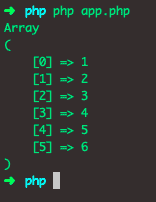# PHP Array Reduce: How to reduce array elements in PHP

PHP array_reduce() method iteratively reduces the array to a single value using a callback function.

## PHP array reduce

PHP array_reduce() is a built-in function that sends the values in the array to a user-defined function and returns a string. The array_reduce() function is used to reduce the elements of an array into a single value that can be of a float, an integer, or a string value. If an array is empty and the initial is not passed, this function returns NULL.

### Syntax

See the below syntax.

`array_reduce(array, myfunction, initial)`

### Arguments

An array parameter is required, and it specifies an array.

The myfunction is required, and it specifies the name of the function.

An initial parameter is required, and it specifies the initial value that needs to send the function.

### Example

See the below code example.

```<?php

// app.php

function rdce(\$v1, \$v2)
{
return \$v1."~~".\$v2;
}

\$arr = ["Emilia","Lena","Kit"];
print_r(array_reduce(\$arr,"rdce"));```

See the below output.Let’s take an example of integers. See the below code.

```<?php

// app.php

function rdce(\$x1, \$x2)
{
return \$x1 + \$x2;
}

\$arr = [19, 21, 46];
print_r(array_reduce(\$arr,"rdce"));```

See the output.It will return the total of the provided integers.

To make it more explicit about what the two parameters of the callback are for, and what “reduce to the single value” actually means (using associative and commutative operators as examples may obscure this).

The first parameter to the callback is an accumulator where the result-in-progress is expertly assembled. If you supply an initial_value the accumulator starts with that value; otherwise, it begins output to Null.
The second parameter is where each value of the array is passed during each step of the reduction.

We can also provide the third argument which counts as an initial value for the reduce function. If you do provide an initial value, the first value used in the iteration is provided value, and then it adds every time until all the array values are iterated.

See the below code example.

```<?php

// app.php

function rdce(\$x1, \$x2, \$x0 = 3)
{
return \$x1 + \$x2 + \$x0;
}

\$arr = [19, 21, 46];
print_r(array_reduce(\$arr, "rdce"));```

In the above code, the sum of the \$arr is 86, but since then there are three items inside the array, and the initial value is 3. So (3 * 3) = 9 will add in the final sum, and the output is 95 in the console.

The cumulative output of the array_reduce() function is 95. See the below output.### Reduce two dimensions to one dimension using array_reduce

You can reduce a two-dimensional array into one-dimensional using array_reduce and array_merge.

See the below code.

```<?php

// app.php

\$two_dimensional = array();
\$two_dimensional['app'] = [1, 2, 3];
\$two_dimensional['dividend'] = [4, 5, 6];
print_r(array_reduce(\$two_dimensional, 'array_merge', array()));```

See the output.That’s it for this tutorial.

Categories PHP

This site uses Akismet to reduce spam. Learn how your comment data is processed.